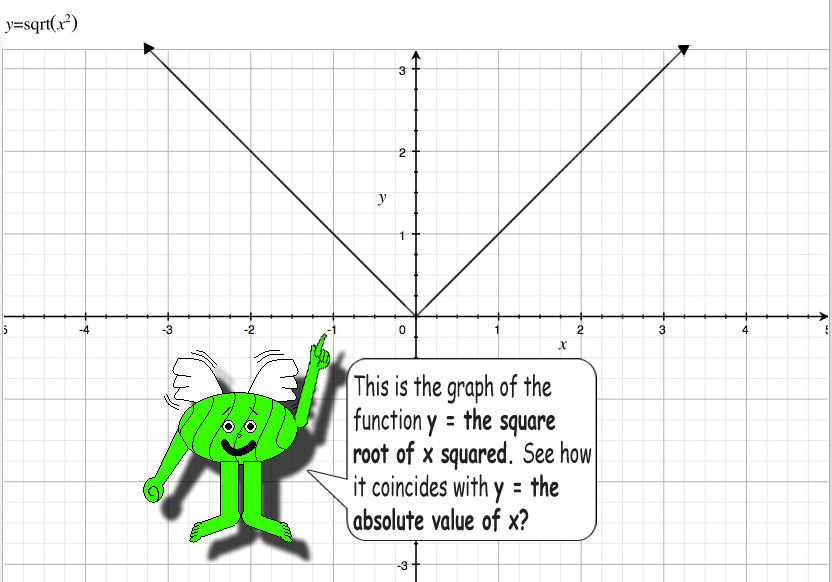# When Square Roots & Absolute Value Match!## Example:

(32)^½ = 9^½ = 3

and

((-3)2)^½ = 9^½ = 3

The caret (^) is the symbol of exponentiation. Raising a number to the power of ½ gives you the square root.

Also, |-3| = 3

### You know that absolute value is always positive, right? Absolute value is also always a real number since imaginary numbers have real absolute values.Squaring a negative number gives you a positive number, but I used another pair of parentheses around the negative three since graphing calculators tend to multiply the square by -1 without the parentheses. That causes some confusion!